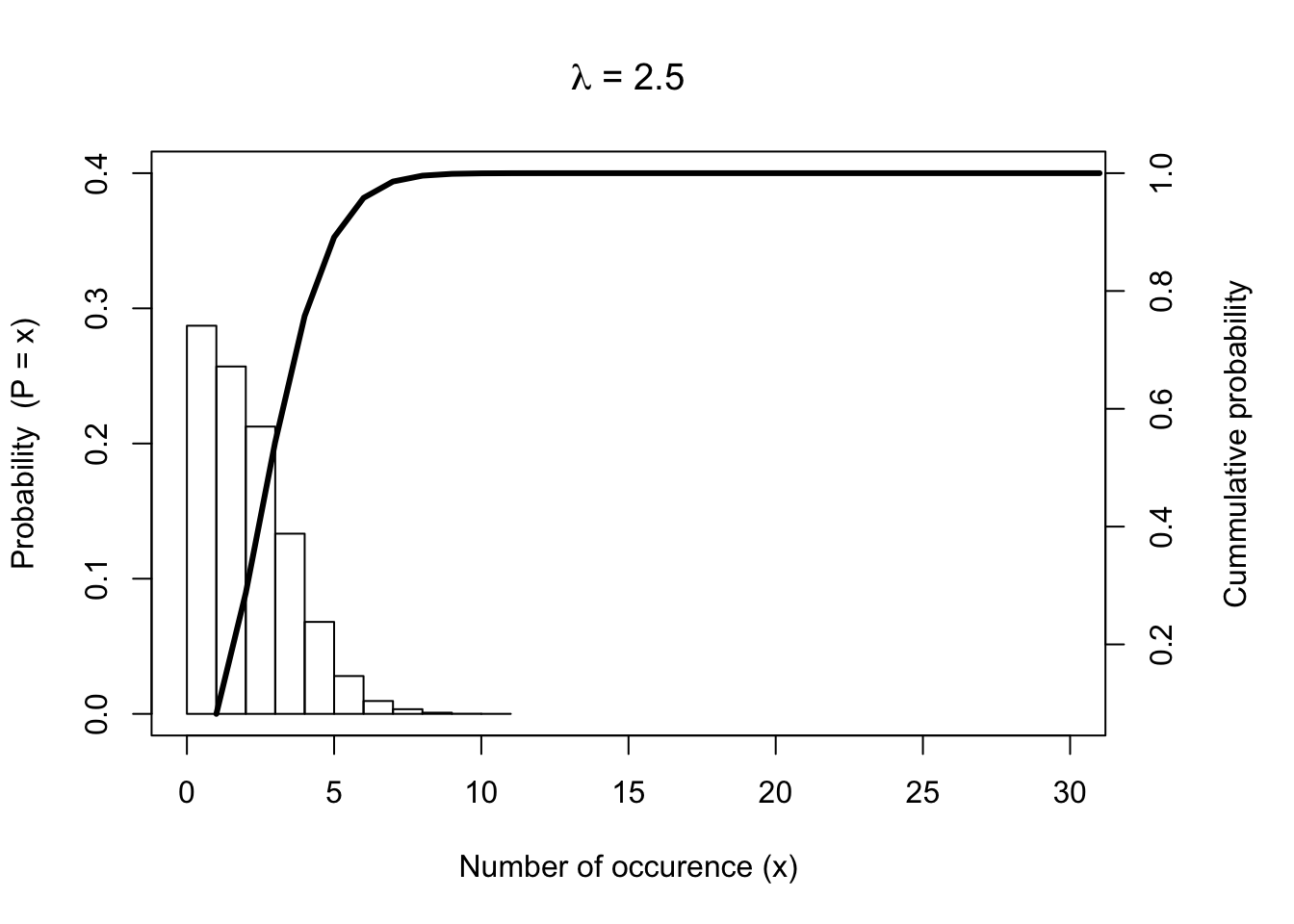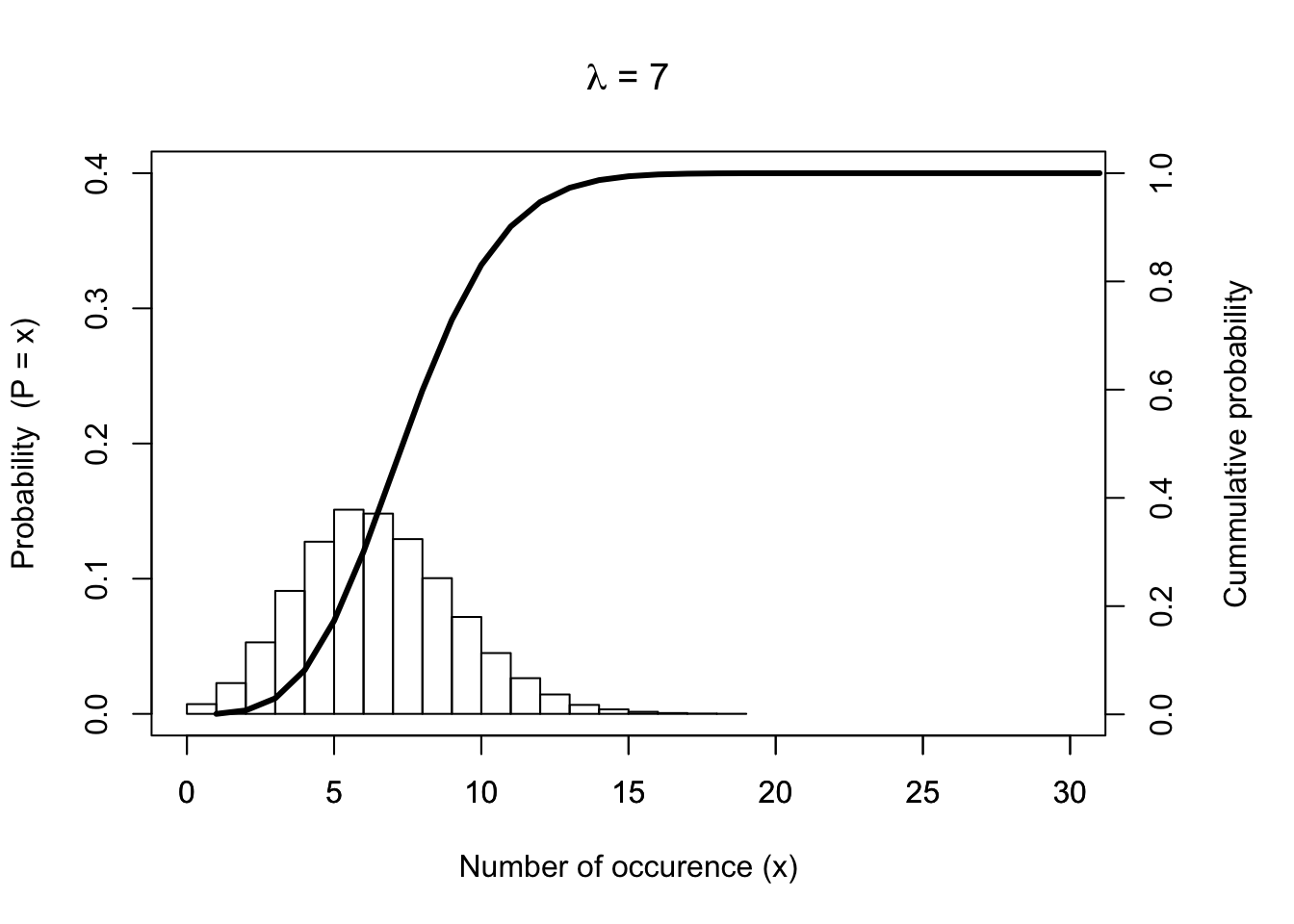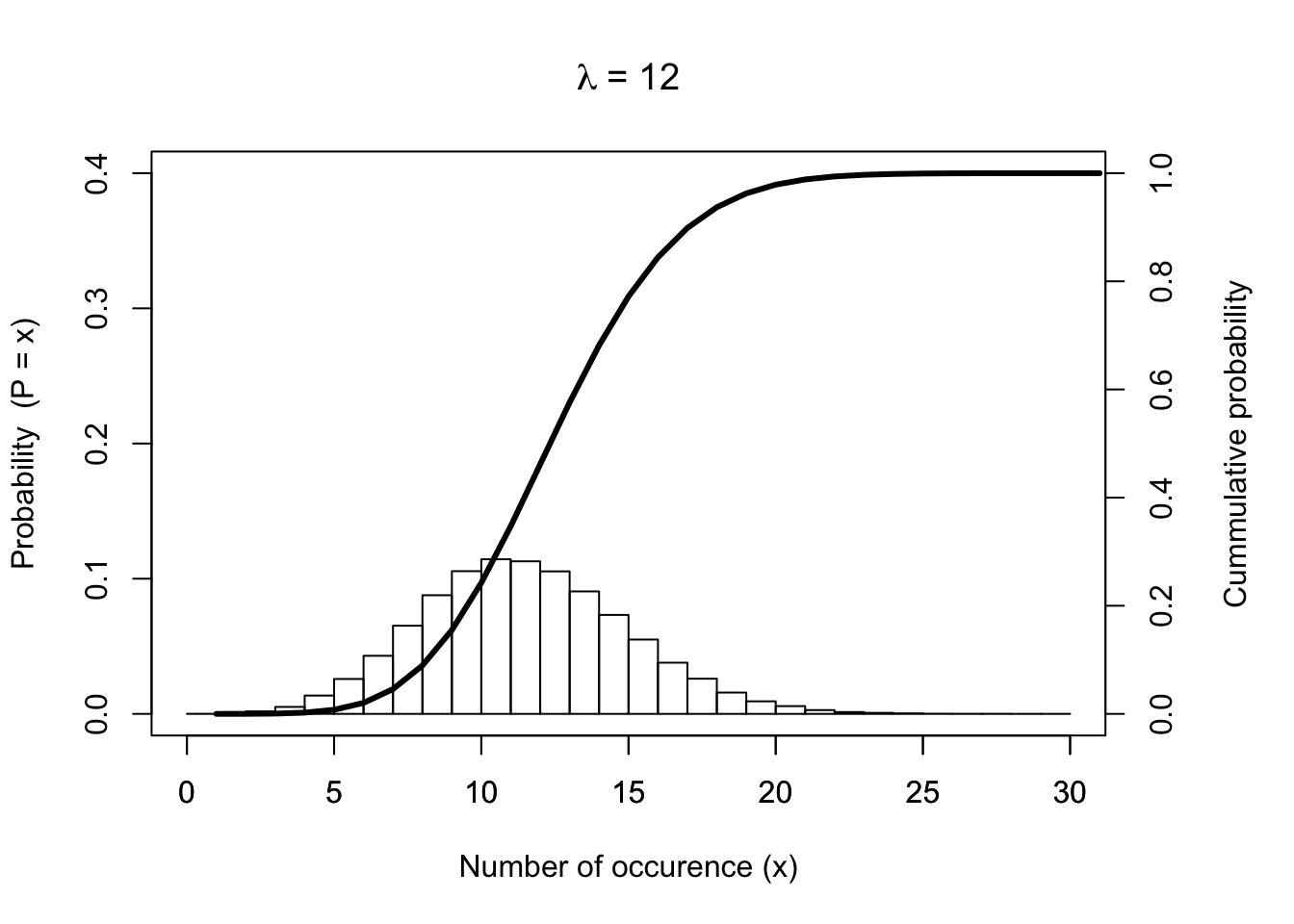For those situations in which $$n$$ is large and $$p$$ is very small, the Poisson distribution can be used to approximate the binomial distribution. Recall the binomial probability distribution:

$P(X = x) = {n \choose x}p^x(1-p)^{n-x}, \qquad x = 0, 1, 2, . . . , n$

As in the “100 year flood” example above, $$n$$ is a large number (100) and $$p$$ is a small number (0.01). Plugging into the equation from above $$P(x = 1)$$ yields

\begin{align} \ P(X = 1) & = {100 \choose 1}\times 0.01^1\times (1 - 0.01)^{100 -1} \\ & = 100\times 0.01\times 0.3697296 \\ & = 0.3697296 \end{align}

The result is very close to the result obtained above dpois(x = 1, lambda = 1) $$= 0.3678794$$. The appropriate Poisson distribution is the one whose mean is the same as that of the binomial distribution; that is, $$\lambda = np$$, which in our example is $$\lambda = 100 \times 0.01 = 1$$.

In order to conclude this section, and in order to give you some intuition of the shapes of different Poisson probability distributions, three different Poisson probability and its corresponding cumulative Poisson probability distributions for $$\lambda =2.5$$, $$\lambda =7$$, $$\lambda = 12$$ are given below.